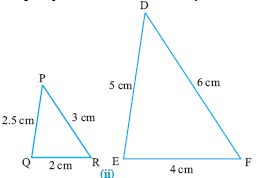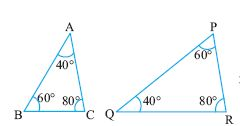Name: ___________________Date:___________________

kwizNET Subscribers, please login to turn off the Ads!
 Email us to get an instant 20% discount on highly effective K-12 Math & English kwizNET Programs!

### Geometry2.23 Properties of Similar Triangles

 Congruency and Similarity: Figures that have same shape and size are said to be congruent, whereas figures need to be of the same shape but not necessarily of the same size for them to be similar. Properties of similar triangles Property 1: If in two triangles, angles are equal, then their corresponding sides are proportional and hence the triangles are similar. This property is called AAA postulate. Property 2: If one angle of two triangle is equal to the other and their sides are proportional, then the triangles are similar by SAS postulate. Property 3: If two angles and one side of two triangles are equal, then they are similar by ASA postulate. Example: In triangle ABC, prove that ABC and DEF are similar.Solution:: In triangles ABC and ADE, we have angle A = angle A (common angle) angle B = angle E (90o) Hence triangles ABC and ADE are similar. Directions: Solve the following problems. Also write at least 5 examples of your own.
 Q 1: Find angle EBA.45o70o60o Q 2: Find angle DEC30o65o45o Q 3: State if the given pair of triangles are congruent and the criterion used?Answer: Q 4: Find angle DCE30o80o45o Q 5: Given two triangles ABC and DEF. AB = 3 cms, angle A = 40, angle D = 40, AC = 5 cms, DE= 4.5 cm, find angle B DF = 7.5 cm30o60o45o Q 6: State if the pair of triangles are congruent and if congruent by what postulate?Answer: Q 7: Find angle EAB60o75o45o Q 8: Find angle AEB30o45o65o Question 9: This question is available to subscribers only! Question 10: This question is available to subscribers only!

#### Subscription to kwizNET Learning System costs less than \$1 per month & offers the following benefits:

• Unrestricted access to grade appropriate lessons, quizzes, & printable worksheets
• Instant scoring of online quizzes
• Progress tracking and award certificates to keep your student motivated
• Unlimited practice with auto-generated 'WIZ MATH' quizzes
• Child-friendly website with no advertisements

© 2003-2007 kwizNET Learning System LLC. All rights reserved. This material may not be reproduced, displayed, modified or distributed without the express prior written permission of the copyright holder. For permission, contact info@kwizNET.com
For unlimited printable worksheets & more, go to http://www.kwizNET.com.